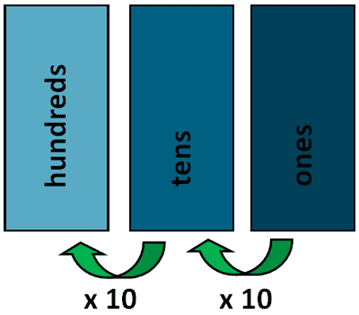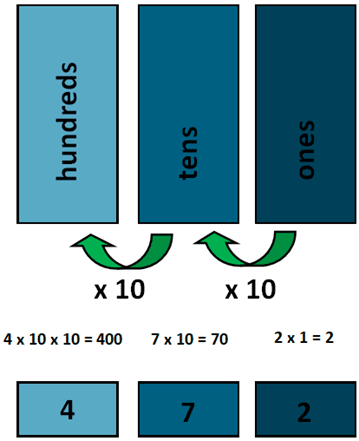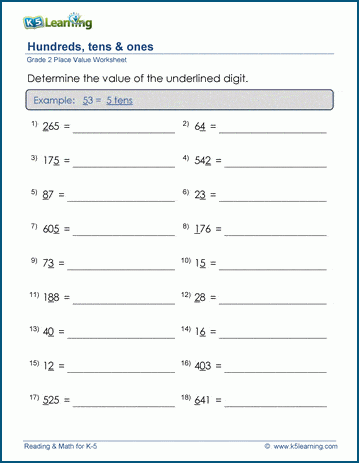The K5 Learning Blog urges parents to be pro-active in helping their children reach their full academic potential.

K5 Learning
provides free worksheets, workbooks and an online reading and math program for kindergarten to grade 5 students.# Place Value Worksheets for 3-Digit Numbers

In grade 2 students learn the place value of 3-digit numbers.

## What is place value?

Place value is the location of a digit in a number. The value of a digit depends on its position in a number. Each place has the value of 10 times the place to its right. For example, the number 68 is made up of 60 and 8.

## 3-digit numbers

In 3-digit numbers, students learning about the positions of ones, tens and hundreds. As you can see, each number has the value 10 times that of the number on its right:Let’s use an example: 472, four hundred seventy two.
There are two ones: 2 x 1 = 2
There are 7 tens: 7 x 10 = 70
There are 4 hundreds: 4 x 100 = 400## Grade 2 place value worksheets

In our grade 2 place value section we have a variety of worksheets that help students practice 3-digit numbers.